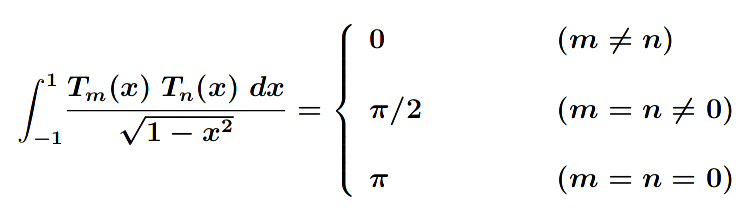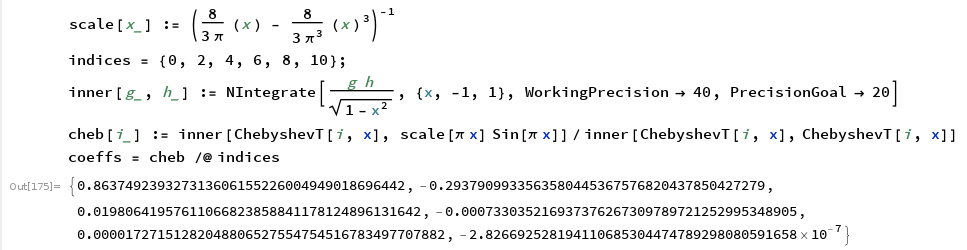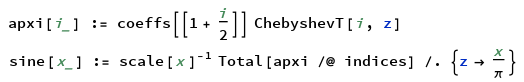# Approximating sin(x) to 5 ULP with Chebyshev polynomials

By Colin Wallace
May 11, 2017 (last revised Mar 18, 2018)
```fn sine(x: f32) -> f32 { let coeffs = [ -0.10132118f32, // x 0.0066208798f32, // x^3 -0.00017350505f32, // x^5 0.0000025222919f32, // x^7 -0.000000023317787f32, // x^9 0.00000000013291342f32, // x^11 ]; let pi_major = 3.1415927f32; let pi_minor = -0.00000008742278f32; let x2 = x*x; let p11 = coeffs; let p9 = p11*x2 + coeffs; let p7 = p9*x2 + coeffs; let p5 = p7*x2 + coeffs; let p3 = p5*x2 + coeffs; let p1 = p3*x2 + coeffs; (x - pi_major - pi_minor) * (x + pi_major + pi_minor) * p1 * x } ```

That code above will compute the sine of any IEEE single-precision float in the range of to within 4.58 Units of Least Precision (ULP). A ULP is a useful way to measure relative error in a floating point computation. Given two adjacent floating point numbers, their difference in terms of ULP is essentially equivalent to the number of floats that lie between them (plus one).

Given that IEEE single-precision floats have 24 bits of precision, this sine function is accurate to within 0.000027% (i.e. ). Achieving this precision over a domain this large was a challenge for me. Many polynomial approximations to operate only over or and use special properties of the sine function to reduce their arguments into this range (e.g. to force arguments into ). To work over larger ranges without reduction (e.g. if the input is known to be bounded by ), these methods require modifications that I had not seen published before, so the remainder of this article will discuss my approach to deriving the sine function above.

When I need to distinguish between the approximation of and the true , I'll be using to represent the approximation.

# Sin(x) as a sum of Chebyshev polynomials

The first step is to re-express over the domain of interest as an infinite polynomial. One could use a Taylor series, but convergence is very slow. Instead, I use Chebyshev polynomials.

For me, it helps to view these things using concepts from linear algebra. Any continuous and smooth function can be expressed as a polynomial: . This is a weighted sum of powers of , and because are all linearly independent, we can think of this set as a basis for our function.

Instead of representing our function in terms of the standard polynomial basis (), we can use , where is the Chebyshev polynomial of the first kind.The first five Chebyshev polynomials. From Wikipedia (public domain).

Chebyshev polynomials have the property that all their local extrema lie within , and each such extrema has a value of exactly . For those familiar with Fourier series, this should look somewhat familiar. We're projecting the sine function onto a basis where each basis function is oscillating at an increasing rate. It's intuitive, then, that the smoother a function is, the more rapidly the coefficients of its projection onto will decay (e.g. doesn't oscillate rapidly over the interval , so any rapidly-oscillating component would have to be small). Although the basis has infinite cardinality, most energy is located in the lower components. We can truncate the basis to for a relatively small value of N and achieve a very good approximation of over the interval .

Note that for a smooth function approximated with a finite set of Chebyshev polynomials, the error is spread in a fairly uniform manner. The approximation isn't intrinsically more accurate near 0 and less accurate at the extremeties, for example. We can say that for all , where is the error of the approximation function (), and is some bound that decreases as we increase the number of terms in the approximation.

This is good. If we approximated with 6 chebyshev terms, we might well get the error bound, , down to . However, optimizing for absolute error is generally not what we want! The nature of floating point numbers is such that precision is as much as near zero, and as little as near one. What we really want to minimize is the relative error. As long as , we know that there is no closer float to the true value than what we've approximated*.

* The catch is that achieving only ensures we're off by less than 1 ULP sans rounding. If the true answer falls in between two floats, this isn't enough to guarantee that we round to the correct one. If the answer is nearly the average of the two adjacent floats, being off by just 0.01 ULP in our model could cause incorrect rounding that actually bumps us to a full 0.5 ULP error. I imagine optimizing for 0.5 ULP error requires more novel techniques. I found a paper detailing Intel's formally-verified sine implementation for their IA-64 architecture, and even it only claims accuracy to 0.57 ULP.

# Optimizing relative error

To optimize for relative error, we first scale by some easily-reversible function in order to make the result have less dynamic range. For example, if we scale by , we get something that looks like the below plot.Scaled sine function with a dynamic range of ~2, plotted on .

The advantage of this is that if we optimize the absolute error of the above function to , where is the dynamic range, then we can apply the inverse scaling function and obtain a approximation that's accurate to a relative error of everywhere. I derived the scaling function by solving the min-degree odd polynomial that has a zero at and , and a one at .

Let's now project the scaled sine function onto the Chebyshev basis polynomials. To do this, I followed pages 7-8 of this University of Waterloo pdf. Specifically, it shows the following property for Chebyshev functions, which arises from their orthogonality.Chebyshev inner-product equality

If the scaled sine function is representable in terms of the Chebyshev basis functions, i.e. , then the integral is exactly equal to . By moving out of the integral, the following relation is obtained: , where represents the inner product, i.e. . This gives a straightforward way to solve for each and thereby re-express the scaled sine function in terms of the Chebyshev basis.

I compute the first 11 terms of the series with the following Mathematica code. I omit solving for and because symmetry requires they be zero. In order to keep the function's domain as , I scale the parameter to by . This way, we get coefficients to a function that computes one full cycle of . I'll undo the scaling of later.Solve for the Chebyshev coefficients to the scaled sine function. WorkingPrecision needs to be raised for NIntegrate to converge (or use Integrate, if you're patient).

Now that we have the Chebyshev coefficients, we can reconstruct an approximation to and also undo the scaling of the parameter:Reconstructing

A quick plot of the reconstructed sine function looks promising:Plot of reconstructed sine approximation on .

Likewise, the plot for the relative error measurement: .Relative error of the sine approximation on .

The error is fairly uniform, and it reaches the target of . For completeness, the polynomial coefficients are shown below. Chebyshev functions are themselves polynomials, so expanding the function gives an ordinary polynomial.Polynomial approximation to .

# Evaluating the polynomial approximation

Given these coefficients, the polynomial can be evaulated with Horner's method (aka "nested multiplication"):

```fn sine(x: f32) -> f32 { let coeffs = [ 0.999999999973088f32, // x -0.1666666663960699f32, // x^3 0.00833333287058762f32, // x^5 -0.0001984123883227529f32, // x^7, 2.755627491096882e-6f32, // x^9 -2.503262029557047e-8f32, // x^11 1.58535563425041e-10f32, // x^13 ]; let p13 = coeffs; let p11 = p13*x*x + coeffs; let p9 = p11*x*x + coeffs; let p7 = p9*x*x + coeffs; let p5 = p7*x*x + coeffs; let p3 = p5*x*x + coeffs; let p1 = p3*x*x + coeffs; p1*x } ```

So how does this perform?Average error (in ULP) v.s. x.

Not very well, but why?

It turns out we've reached the point where the low-error approximation is only accurate when evaluated with infinite precision. When we perform the computations using 32-bit floats, we're plagued by rounding errors. You see that first coefficient: 0.999999999973088f32?

```> println!("{}", 0.999999999973088f32 == 1.0f32); true ```

Yup, we chose coefficients that can't even be represented in our number system. Of course the results have error. As for what we can do about it, notice where the error occurs. It only becomes excessive as approaches , at which point should approach 0. But the slight bit of error in the coefficients makes it so it doesn't approach 0 at quite the right place. Our polynomial already has a factor of explicitly factored in order to create a root at . My instincts are to pull out a factor of and as well.approximation divided by .

Now if I multiplied by in 32-bit float to undo the division, that wouldn't be quite right, because the float closest to isn't exactly equal to the true value of . The result is that sin(f32::consts::PI) shouldn't be 0, so we don't want a root at (x-f32::consts::PI).

Instead, I'll make a root at , where is the closest f32 to the real , and is a small correction factor that's approximately the true minus the f32 . Because the correction factor, , is close to 0, the net value being added/subtracted is accurate to more decimal places. The effect is only seen when x is near , otherwise the offset itself gets absorbed by rounding errors. But that's ok, because it's only needed to fix errors near in the first place.

```let coeffs = [ -0.101321183346709072589001712988183609230944236760490476f32, // x 0.00662087952180793343258682906697112938547424931632185616f32, // x^3 -0.000173505057912483501491115906801116298084629719204655552f32, // x^5 2.52229235749396866288379170129828403876289663605034418e-6f32, // x^7 -2.33177897192836082466066115718536782354224647348350113e-8f32, // x^9 1.32913446369766718120324917415992976452154154051525892e-10f32, // x^11 ]; let x2 = x*x; let p11 = coeffs; let p9 = p11*x2 + coeffs; let p7 = p9*x2 + coeffs; let p5 = p7*x2 + coeffs; let p3 = p5*x2 + coeffs; let p1 = p3*x2 + coeffs; (x - 3.1415927410125732f32 + 0.00000008742277657347586f32) * (x + 3.1415927410125732f32 - 0.00000008742277657347586f32) * p1 * x ```Average error (in ULP) v.s. x.

At this point, it helps to look at the error bounds, rather than just their average magnitude:Max & min error (signed) v.s. x. The global maximum error is 4.90 ULP, at x=2.6183214.

The plot above shows that the error oscillates over a large range, and rapidly. The plot was made by taking the min/max signed error over each group of 4096 adjacent floats, so we see that the signed error changes by as much as 9 ULP over the course of just 4096 adjacent floats. It doesn't seem that the error could be meaningfully addressed by adding more polynomial terms or better optimization of the existing polynomial coefficients, then - it's essentially noise.

Without resorting to piecewise functions (or another form of control logic), I think my options are fairly limited at this point. I could use a similar trick as with subtracting , and represent each coefficient more precisely as a sum of two floats with differing magnitude (i.e. the f32 analog of double doubles), but at the cost of more complexity. Or I could brute-force search nearby sets of coefficients and see if there are any that just happen to be less susceptible to this noise.

So I ran the brute-forcer for 20 hours and it turned up a slightly better result (it actually got this result within the first hour). The resulting coefficients are the ones shown in the code at the top of this article. The maximum error over is now 4.58 ULP and occurs at x=3.020473.Max & min error v.s. x for the brute-forced coefficients.

Although performance wasn't my goal here, I'll conclude with a benchmark against the standard library's implementation, which probably handles infinities, NaNs, and performs reduction for numbers outside .

```extern crate rand; extern crate test; use rand::{Rng, XorShiftRng}; use test::Bencher; const TWO_PI: f32 = 2f32*std::f32::consts::PI; #[bench] fn bench_my_sin(b: &mut Bencher) { let mut rng = XorShiftRng::new_unseeded(); b.iter(|| { let x = (rng.next_f32()-0.5f32) * TWO_PI; bruteforce_sine(x) }); } #[bench] fn bench_f32_sin(b: &mut Bencher) { let mut rng = XorShiftRng::new_unseeded(); b.iter(|| { let x = (rng.next_f32()-0.5f32) * TWO_PI; x.sin() }); } ```
```\$ cargo bench test bench_f32_sin ... bench: 16 ns/iter (+/- 1) test bench_my_sin ... bench: 7 ns/iter (+/- 1) ```

# Extras

A literal adaptation of this code to compute instead of is provided below (this change to "normalized frequency" is commonly required in DSP). The maximum error is 4.79 ULP. ```let coeffs = [ -3.1415926444234477f32, // x 2.0261194642649887f32, // x^3 -0.5240361513980939f32, // x^5 0.0751872634325299f32, // x^7 -0.006860187425683514f32, // x^9 0.000385937753182769f32, // x^11 ]; let x2 = x*x; let p11 = coeffs; let p9 = p11*x2 + coeffs; let p7 = p9*x2 + coeffs; let p5 = p7*x2 + coeffs; let p3 = p5*x2 + coeffs; let p1 = p3*x2 + coeffs; (x-1f32) * (x+1f32) * p1 * x ```